# Vector Product of Vectors

The vector product and the scalar product are the two ways of multiplying vectors which see the most application in physics and astronomy. The magnitude of the vector product of two vectors can be constructed by taking the product of the magnitudes of the vectors times the sine of the angle (<180 degrees) between them. The magnitude of the vector product can be expressed in the form: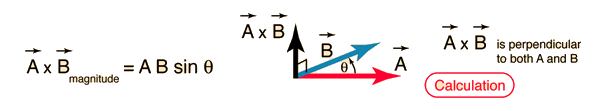and the direction is given by the right-hand rule. If the vectors are expressed in terms of unit vectors i, j, and k in the x, y, and z directions, then the vector product can be expressed in the rather cumbersome form: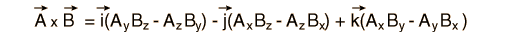which may be stated somewhat more compactly in the form of a determinant.

Index

Vector concepts

Applications

 HyperPhysics***** Mechanics R Nave
Go Back

# Vector Product, Determinant Form

The vector product is compactly stated in the form of a determinant which for the 3x3 case has a convenient evaluation procedure: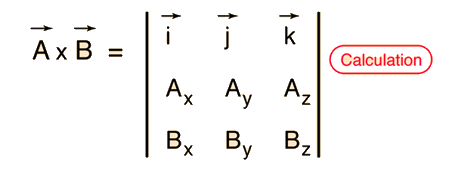Once the scheme for determinant evaluation is familiar, this is a convenient way to reconstruct the expanded form:Index

Vector concepts

 HyperPhysics***** Mechanics R Nave
Go Back

# Vector Product Calculation

You may enter values in any of the boxes below. Then click on the symbol for either the vector product or the angle.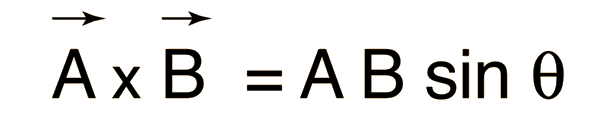The vector product = ( )( )(sin ) degrees.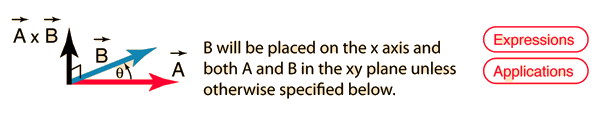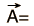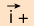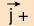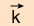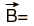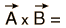magnitude.
Index

Vector concepts

 HyperPhysics***** Mechanics R Nave
Go Back

# Vector Product Applications

Geometrically, the vector product is useful as a method for constructing a vector perpendicular to a plane if you have two vectors in the plane.

Physically, it appears in the calculation of torque and in the calculation of the magnetic force on a moving charge.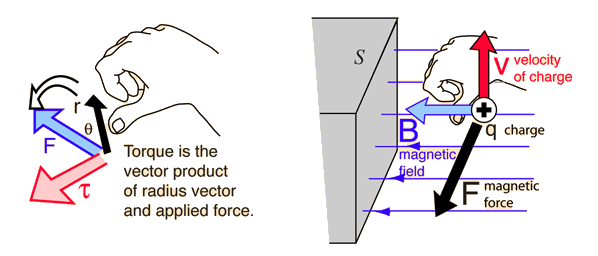Index

Vector concepts

 HyperPhysics***** Mechanics R Nave
Go Back

# Right Hand Rule, Vector Product

The direction of the vector product can be visualized with the right-hand rule. If you curl the fingers of your right hand so that they follow a rotation from vector A to vector B, then the thumb will point in the direction of the vector product.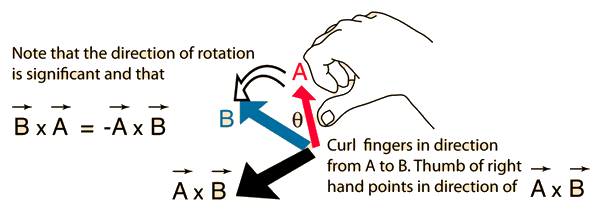The vector product of A and B is always perpendicular to both A and B. Another way of stating that is to say that the vector product is perpendicular to the plane formed by vectors A and B. This right-hand rule direction is produced mathematically by the vector product expression.

Index

Vector concepts

 HyperPhysics***** Mechanics R Nave
Go Back